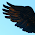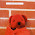## Tuesday, December 21, 2010

I’m in the midst of (yet again) trying to understand what Kant means by (to use the modern spelling) Konstruktion. I first had the thought, which no doubt I’ve had before, that it means “construal,” in the sense of “interpretation.”

That would fit a context such as KdrV A224/B271, where Kant equates “[den Begriff eines Triangels] konstruieren” (to construct/construe the concept of a triangle) with “to give [the concept] … an object,” i.e. to give it what he elsewhere calls “a reference [Beziehung] to objects, and hence a significance [Bedeutung]” (A145–6/B185), or, taking Bedeutung more broadly: to give it an “imagined significance [eingebildete Bedeutung]” (A84/B117), as opposed to a mere “logical significance” (A147/B186). To construe, that is, interpret, is to give significance.

Unfortunately, this appears not to be an accepted sense of the German verb (and moreover, according to the OED, is only a late and derivative sense of the English one). Moreover, we have to take into account another use of konstruieren in the exact same passage (from the proof of the First Postulate). Kant also talks there about the synthesis “wodurch wir in der Einbildungskraft einen Triangle konstruieren” (A224/B271). Whatever that means, it doesn’t mean “by which we construe (= interpret) a triangle in the imagination.”

Many would take it, instead, to mean something like: by which we construct (= build up) a triangle out of imaginary pieces, using an imaginary compass and an imaginary ruler, on a piece of imaginary paper. But that in itself makes little sense. Moreover, to the extent that it does make sense, it leads straight back to Descartes’s argument that we can’t do any such imaginary drawing in the case of a chiliagon (a point of which Kant is well aware: see A140/B179–80). Finally, such an understanding of konstruieren wouldn’t work at all in the first example, where Kant talks of constructing the concept of a triangle. A concept presumably can’t be drawn on any paper, no matter how imaginary.

So my new thought is this: that both of these ways of talking are short for: “einen Begriff mit einem Gegenstand konstruieren,” to construe a concept with an object (on the model of: to construe a verb with an object).

If we can construe a concept with an object (via a schema), then we know that it is at least the concept of an ens imaginarium (not, like the concept diangle, that of a nihil negativum [A291/B348]; and not, of course, of an ens rationis or nihil privativum, either). What he’s saying in the proof of the First Postulate (i.e., the proof that “what agrees with the formal conditions of experience (according to intuitions and concepts) is possible” [A218/B265]) is that to promote a concept from the concept of an ens imaginarium (which, although not impossible, is still a kind of nothing) to the concept of a possible being, we need to connect the procedure of the imagination in the schema of that concept to the synthesis of the imagination in perception.

So the object of the concept triangle would remain “always only a product of the imagination” if it were not that

The very same [eben dieselbe] imaging synthesis by which we construe a triangle [with its concept] in the imagination is wholly the same as [mit … einerlei] that which we exercise in the apprehension of an appearance, in order to make from it an empirical concept. (again, A224/B271)

The concept triangle passes this test, but the concepts of pure time and pure space as such, “die zwar Etwas sind, als Formen anzuschauen, aber selbst keine Gegenstände sind, die angeschaut werden” [A291/B347], do not. The synthesis by which the pure imagination produces time and space as pure images of the categories is not the same as any synthesis of the empirical imagination in perception (but is rather a condition of possibility of all such empirical synthesis). Hence the concepts of time and space are concepts of entia imaginaria.1

However, I’m not sure yet if this will work in other places or whether Kant anywhere actually uses the locution “to construe a concept with an object.”

Footnotes

1 The ursprünglich pure intuitions of time and space, I take it, do not have objects: intuitions without concepts are blind.

1.Are you saying that ‘Konstruieren' is a sort of ‘taking’? I suppose I don't quite understand the grammar of “construing a concept with an object.”

2.I'm not sure I have the grammar of it exactly right. It's meant to be related to the DWDS definition of konstruieren as "Satzglieder nach den Regeln der Syntax zu einem Satz zusammenfügen," and to the OED definition of "construe" as "To combine (words, or parts of speech) grammatically. Now, to combine a verb, adjective, preposition, or other word with the case or relational words with which it is syntactically used." They both give examples like "this verb is construed with the dative," but maybe "with" isn't what I want here. Anyway, the idea is that we can associate the concept, so to speak grammatically, with an object. The "grammar" in question is the procedure of the imagination in schematizing the concept, "as a rule of determination of our intuition conformable to [gemäß] a certain universal concept" (A141/B180).

3.This comment has been removed by the author.

4.BTW I should point out that, in addition to the mathematical way of construing/constructing a concept, there is also a dynamical way (see MAdN 4:493).